# Condensed Java 03 Mar21 Python and Java n

• Slides: 52
Download presentation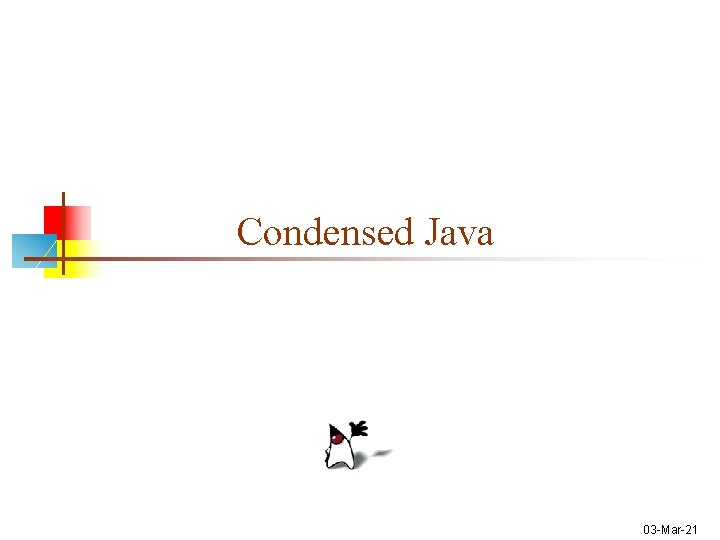Condensed Java 03 -Mar-21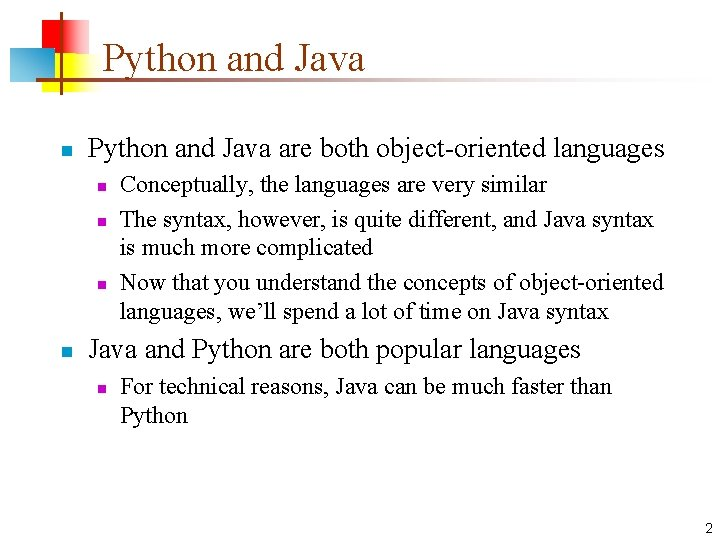Python and Java n Python and Java are both object-oriented languages n n Conceptually, the languages are very similar The syntax, however, is quite different, and Java syntax is much more complicated Now that you understand the concepts of object-oriented languages, we’ll spend a lot of time on Java syntax Java and Python are both popular languages n For technical reasons, Java can be much faster than Python 2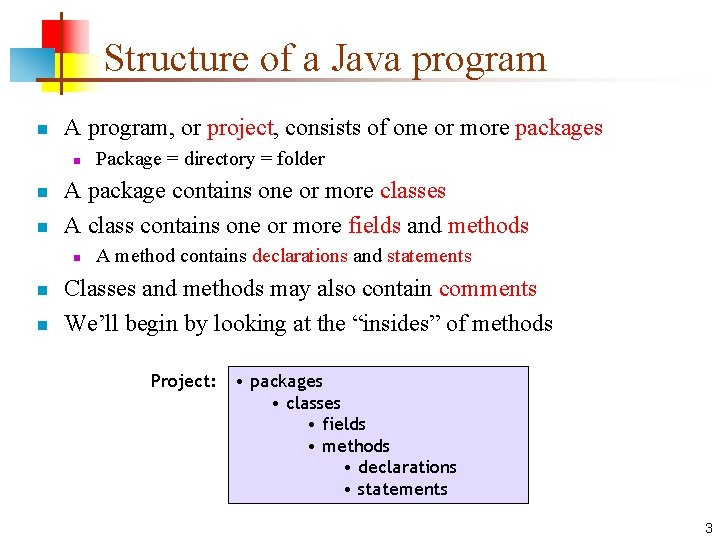Structure of a Java program n A program, or project, consists of one or more packages n n n A package contains one or more classes A class contains one or more fields and methods n n n Package = directory = folder A method contains declarations and statements Classes and methods may also contain comments We’ll begin by looking at the “insides” of methods Project: • packages • classes • fields • methods • declarations • statements 3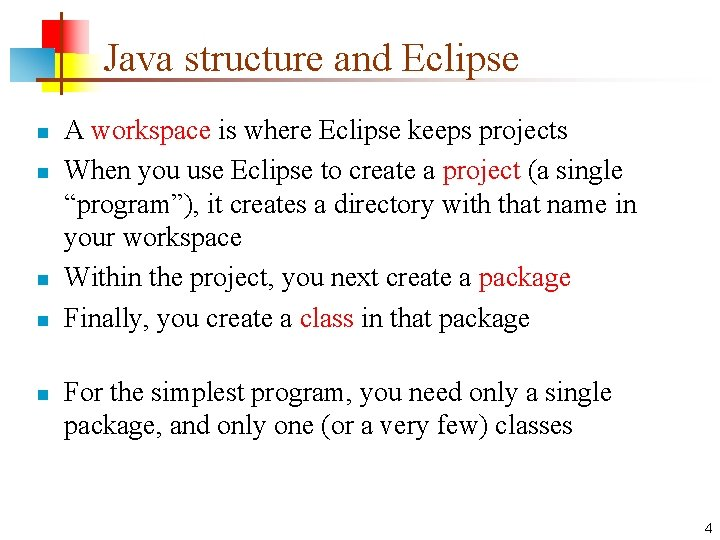Java structure and Eclipse n n n A workspace is where Eclipse keeps projects When you use Eclipse to create a project (a single “program”), it creates a directory with that name in your workspace Within the project, you next create a package Finally, you create a class in that package For the simplest program, you need only a single package, and only one (or a very few) classes 4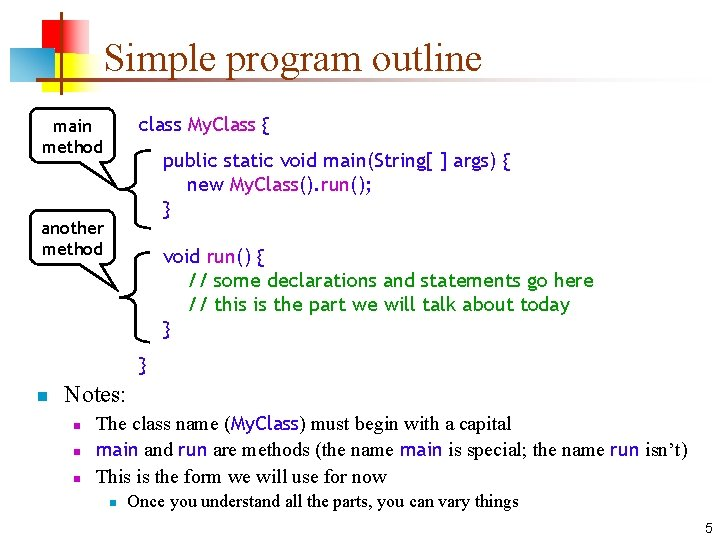Simple program outline class My. Class { main method public static void main(String[ ] args) { new My. Class(). run(); } another method void run() { // some declarations and statements go here // this is the part we will talk about today } } n Notes: n n n The class name (My. Class) must begin with a capital main and run are methods (the name main is special; the name run isn’t) This is the form we will use for now n Once you understand all the parts, you can vary things 5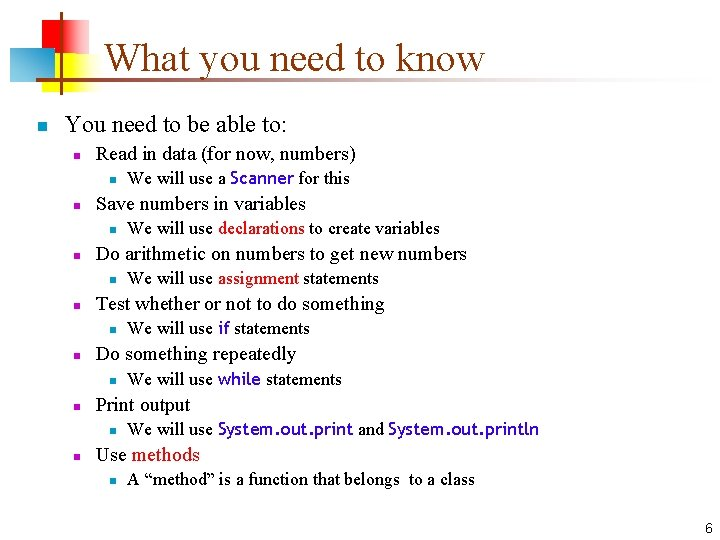What you need to know n You need to be able to: n Read in data (for now, numbers) n n Save numbers in variables n n We will use while statements Print output n n We will use if statements Do something repeatedly n n We will use assignment statements Test whether or not to do something n n We will use declarations to create variables Do arithmetic on numbers to get new numbers n n We will use a Scanner for this We will use System. out. print and System. out. println Use methods n A “method” is a function that belongs to a class 6Declarations, statements, comments n A declaration gives type information to the computer n You must declare: n n A statement tells the computer to do something n n n The type of value (int, String, etc. ) each variable can hold The type of every parameter to a method The type returned by every method Statements should really be called “commands” Statements may only occur within methods Comments are ignored by the compiler n As in Python, there are different comments for people who use your methods, and those who read your code 7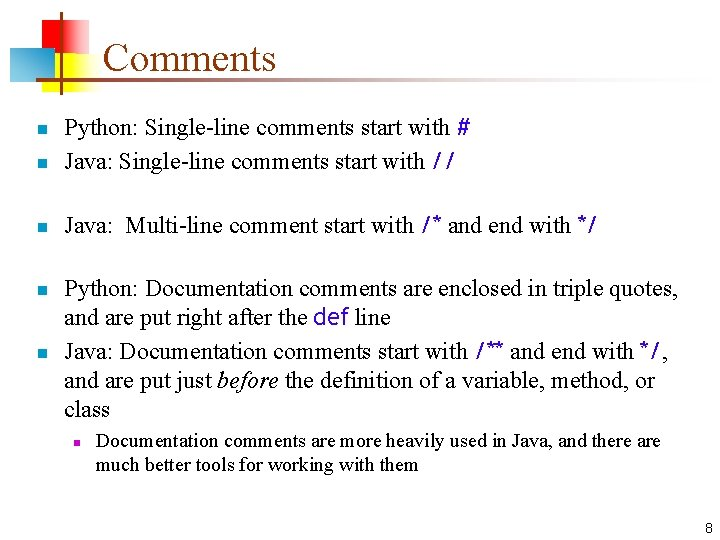Comments n Python: Single-line comments start with # Java: Single-line comments start with // n Java: Multi-line comment start with /* and end with */ n n n Python: Documentation comments are enclosed in triple quotes, and are put right after the def line Java: Documentation comments start with /** and end with */, and are put just before the definition of a variable, method, or class n Documentation comments are more heavily used in Java, and there are much better tools for working with them 8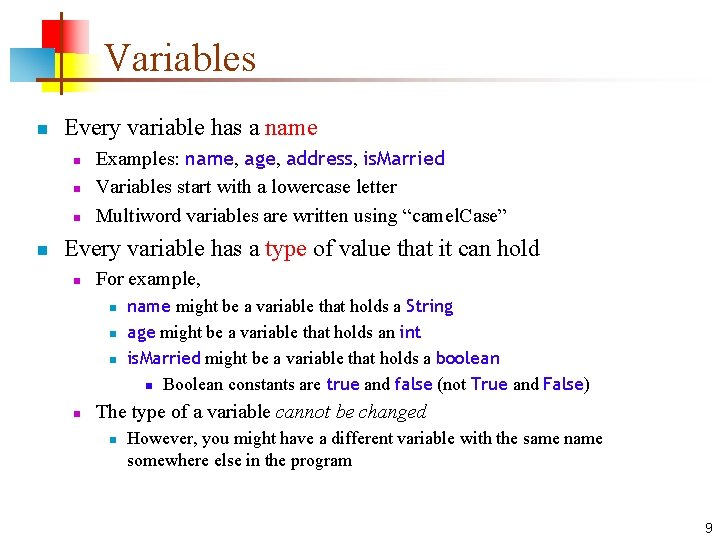Variables n Every variable has a name n n Examples: name, age, address, is. Married Variables start with a lowercase letter Multiword variables are written using “camel. Case” Every variable has a type of value that it can hold n For example, n n name might be a variable that holds a String age might be a variable that holds an int is. Married might be a variable that holds a boolean n Boolean constants are true and false (not True and False) The type of a variable cannot be changed n However, you might have a different variable with the same name somewhere else in the program 9Some Java data types n In Java, the most important primitive (simple) types are: n n Other primitive types are n n int variables hold integer values double variables hold floating-point numbers (numbers containing a decimal point) boolean variables hold a true or false value char variables hold single characters float variables hold less accurate floating-point numbers byte, short and long hold integers with fewer or more digits Another important type is the String n n A String is an Object, not a primitive type A String is composed of zero or more chars 10Declaring variables n n In Python, a variable may hold a value of any type Every variable that you use in a program must be declared (in a declaration) n n n The declaration specifies the type of the variable The declaration may give the variable an initial value Examples: n n n int age; int count = 0; double distance = 37. 95; boolean is. Read. Only = true; String greeting = "Welcome to CIT 591"; String output. Line; 11Multiple values n n An array lets you associate one name with a fixed (but possibly large) number of values Arrays are like Python’s lists, but much less flexible All values must have the same type The values are distinguished by a numerical index between 0 and array size minus 1 0 1 my. Array 12 43 2 6 3 4 5 6 7 8 9 83 14 -57 109 12 0 6 12Using array elements 0 1 my. Array 12 43 n 2 6 3 4 5 6 7 8 9 83 14 -57 109 12 0 6 Examples: • • x = my. Array; my. Array = 99; m = 5; y = my. Array[m]; z = my. Array]; // sets x to 43 // replaces 14 with 99 // sets y to -57 // sets z to 109 13Declaration and definition n To declare an array is to tell its type, but not its size n n To define an array is to give its size n n Example: scores = new int; Declaration and definition can be combined n n Example: int[] scores; Example: int[] scores = new int; The initial content of an array is zero (for numbers), false (for booleans), or null (for objects) 14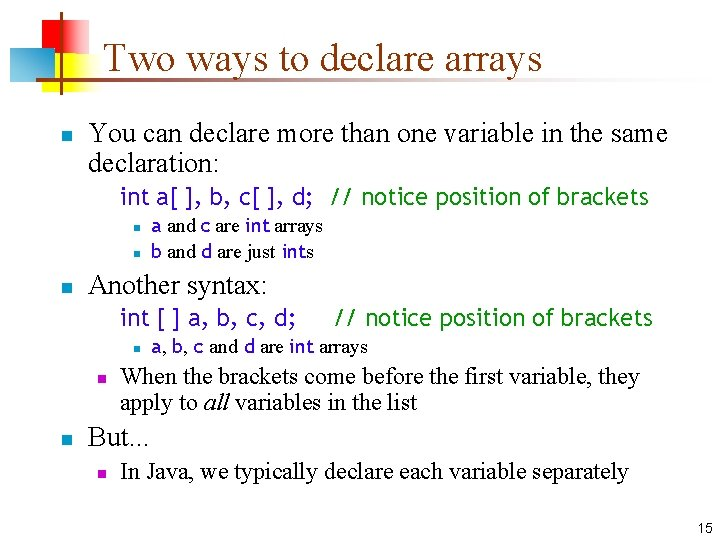Two ways to declare arrays n You can declare more than one variable in the same declaration: int a[ ], b, c[ ], d; // notice position of brackets n n n a and c are int arrays b and d are just ints Another syntax: int [ ] a, b, c, d; n n n // notice position of brackets a, b, c and d are int arrays When the brackets come before the first variable, they apply to all variables in the list But. . . n In Java, we typically declare each variable separately 15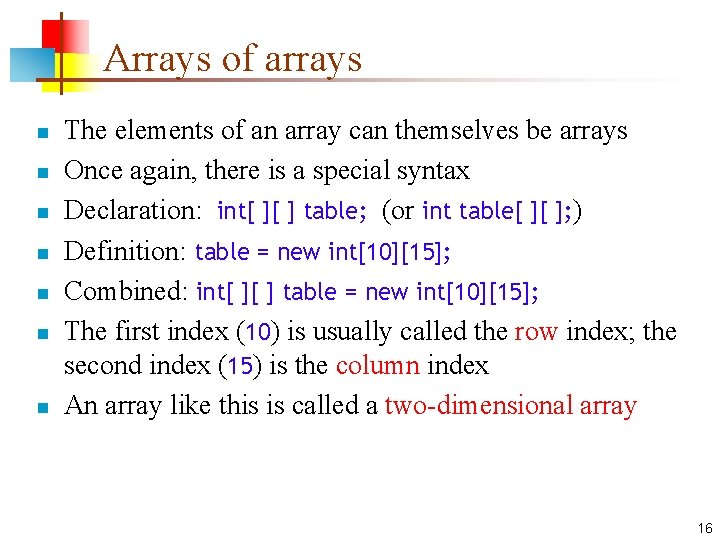Arrays of arrays n n n n The elements of an array can themselves be arrays Once again, there is a special syntax Declaration: int[ ][ ] table; (or int table[ ][ ]; ) Definition: table = new int; Combined: int[ ][ ] table = new int; The first index (10) is usually called the row index; the second index (15) is the column index An array like this is called a two-dimensional array 16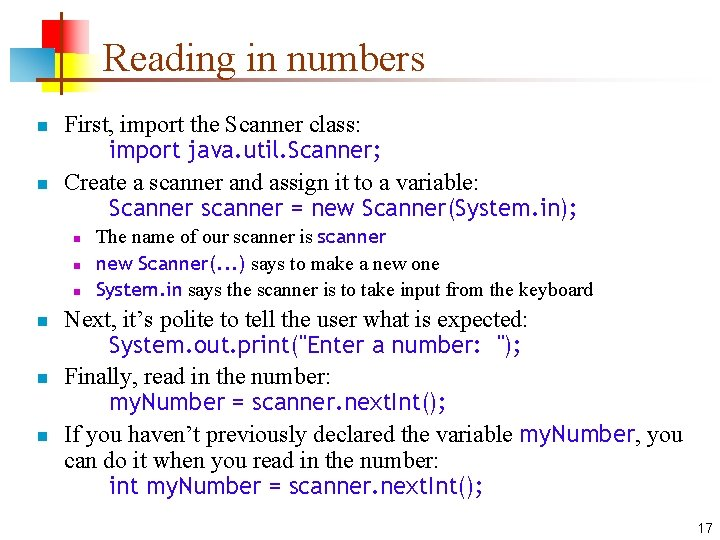Reading in numbers n n First, import the Scanner class: import java. util. Scanner; Create a scanner and assign it to a variable: Scanner scanner = new Scanner(System. in); n n n The name of our scanner is scanner new Scanner(. . . ) says to make a new one System. in says the scanner is to take input from the keyboard Next, it’s polite to tell the user what is expected: System. out. print("Enter a number: "); Finally, read in the number: my. Number = scanner. next. Int(); If you haven’t previously declared the variable my. Number, you can do it when you read in the number: int my. Number = scanner. next. Int(); 17Printing n There are two methods you can use for printing: n System. out. println(something); n n System. out. print(something); n n This prints something and ends the line This prints something and doesn’t end the line (so the next thing you print will go on the same line) These methods will print any one thing, but only one at a time You can concatenate Strings with the + operator Anything concatenated with a String is automatically converted to a String n Example: System. out. println("There are " + apple. Count + " apples and " + orange. Count + " oranges. "); 18Program to double a number n import java. util. Scanner; public class Doubler { public static void main(String[] args) { new Doubler(). run(); } private void run() { Scanner scanner; int number; int doubled. Number; } } scanner = new Scanner(System. in); System. out. print("Enter a number: "); number = scanner. next. Int(); doubled. Number = 2 * number; System. out. println("Twice " + number + " is " + doubled. Number); 19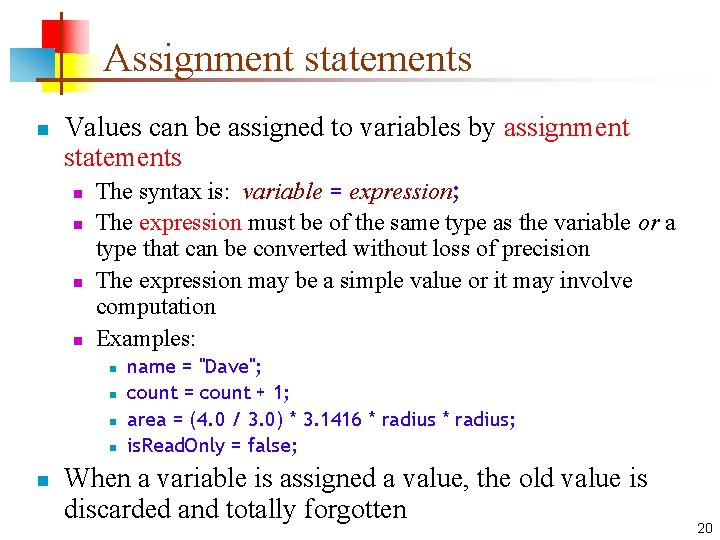Assignment statements n Values can be assigned to variables by assignment statements n n The syntax is: variable = expression; The expression must be of the same type as the variable or a type that can be converted without loss of precision The expression may be a simple value or it may involve computation Examples: n n name = "Dave"; count = count + 1; area = (4. 0 / 3. 0) * 3. 1416 * radius; is. Read. Only = false; When a variable is assigned a value, the old value is discarded and totally forgotten 20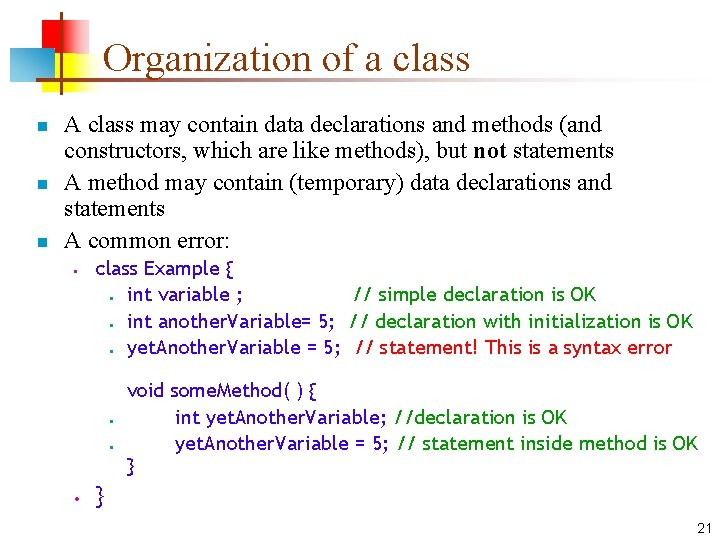Organization of a class n n n A class may contain data declarations and methods (and constructors, which are like methods), but not statements A method may contain (temporary) data declarations and statements A common error: • class Example { • int variable ; // simple declaration is OK • int another. Variable= 5; // declaration with initialization is OK • yet. Another. Variable = 5; // statement! This is a syntax error • • • void some. Method( ) { int yet. Another. Variable; //declaration is OK yet. Another. Variable = 5; // statement inside method is OK } } 21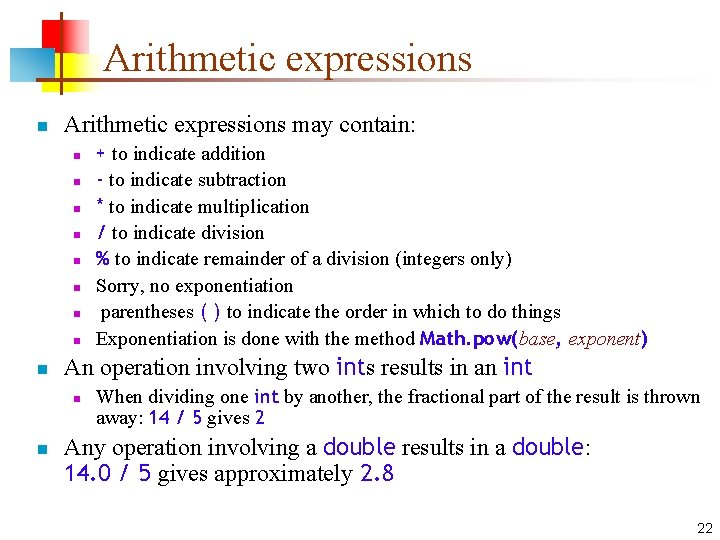Arithmetic expressions n Arithmetic expressions may contain: n n n n n An operation involving two ints results in an int n n + to indicate addition - to indicate subtraction * to indicate multiplication / to indicate division % to indicate remainder of a division (integers only) Sorry, no exponentiation parentheses ( ) to indicate the order in which to do things Exponentiation is done with the method Math. pow(base, exponent) When dividing one int by another, the fractional part of the result is thrown away: 14 / 5 gives 2 Any operation involving a double results in a double: 14. 0 / 5 gives approximately 2. 8 22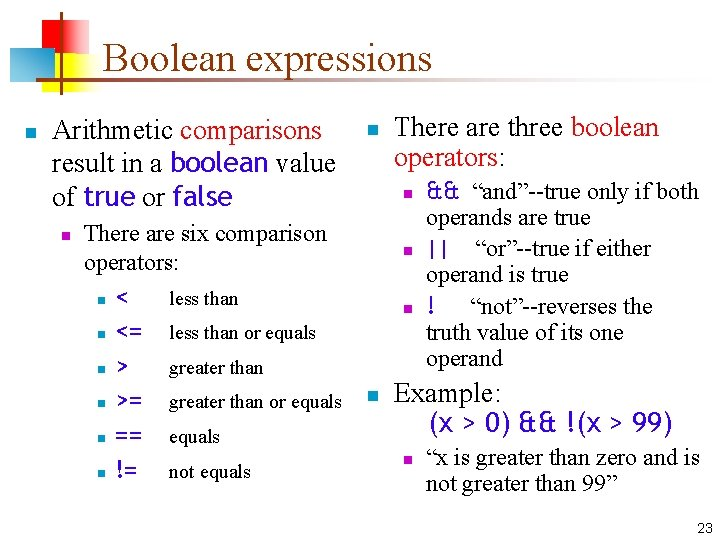Boolean expressions n Arithmetic comparisons result in a boolean value of true or false n There are six comparison operators: n < less than n <= less than or equals n > greater than n >= greater than or equals n == equals n != not equals n There are three boolean operators: n n && “and”--true only if both operands are true || “or”--true if either operand is true ! “not”--reverses the truth value of its one operand Example: (x > 0) && !(x > 99) n “x is greater than zero and is not greater than 99” 23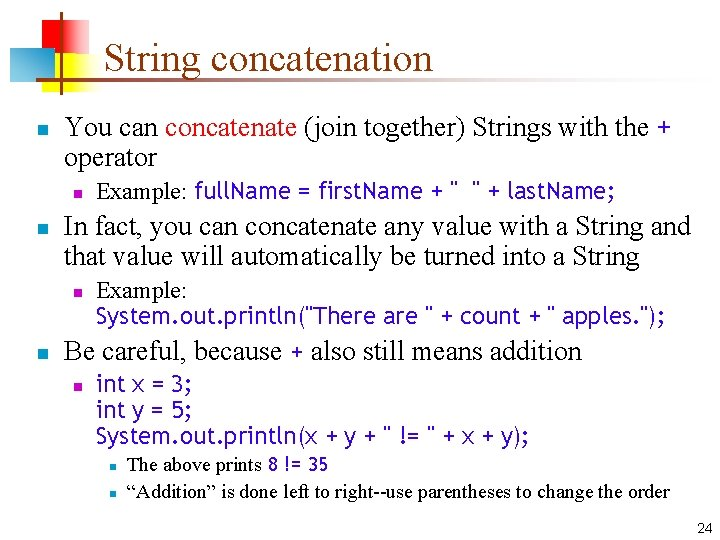String concatenation n You can concatenate (join together) Strings with the + operator n n In fact, you can concatenate any value with a String and that value will automatically be turned into a String n n Example: full. Name = first. Name + " " + last. Name; Example: System. out. println("There are " + count + " apples. "); Be careful, because + also still means addition n int x = 3; int y = 5; System. out. println(x + y + " != " + x + y); n n The above prints 8 != 35 “Addition” is done left to right--use parentheses to change the order 24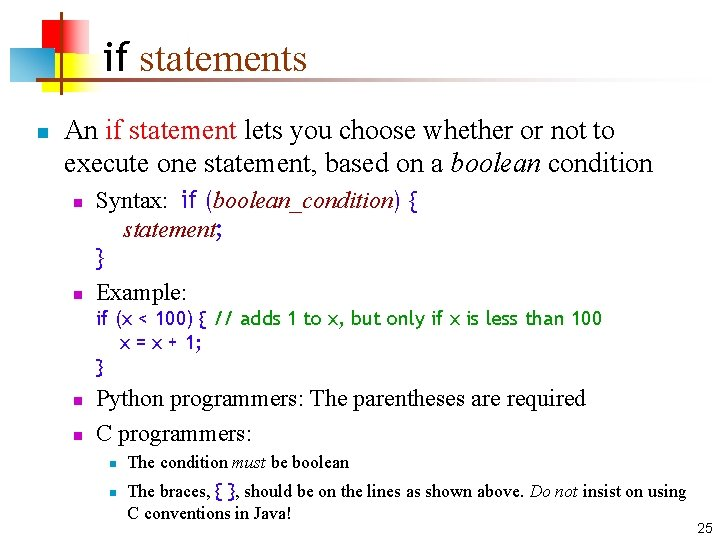if statements n An if statement lets you choose whether or not to execute one statement, based on a boolean condition n n Syntax: if (boolean_condition) { statement; } Example: if (x < 100) { // adds 1 to x, but only if x is less than 100 x = x + 1; } n n Python programmers: The parentheses are required C programmers: n n The condition must be boolean The braces, { }, should be on the lines as shown above. Do not insist on using C conventions in Java! 25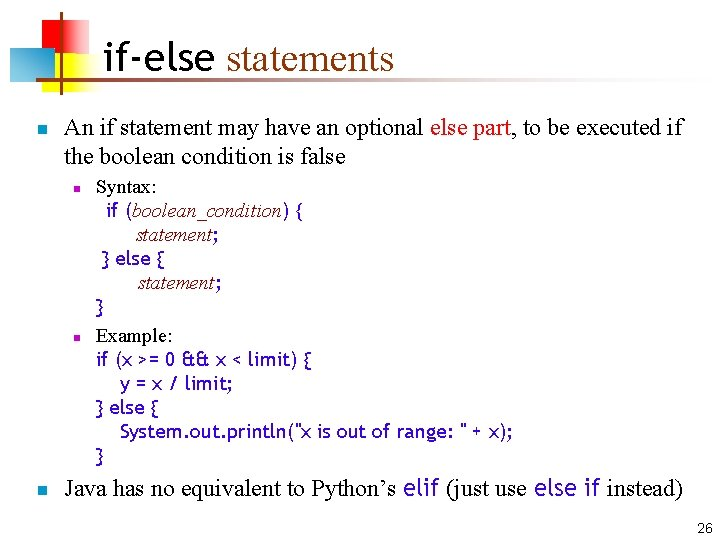if-else statements n An if statement may have an optional else part, to be executed if the boolean condition is false n n n Syntax: if (boolean_condition) { statement; } else { statement; } Example: if (x >= 0 && x < limit) { y = x / limit; } else { System. out. println("x is out of range: " + x); } Java has no equivalent to Python’s elif (just use else if instead) 26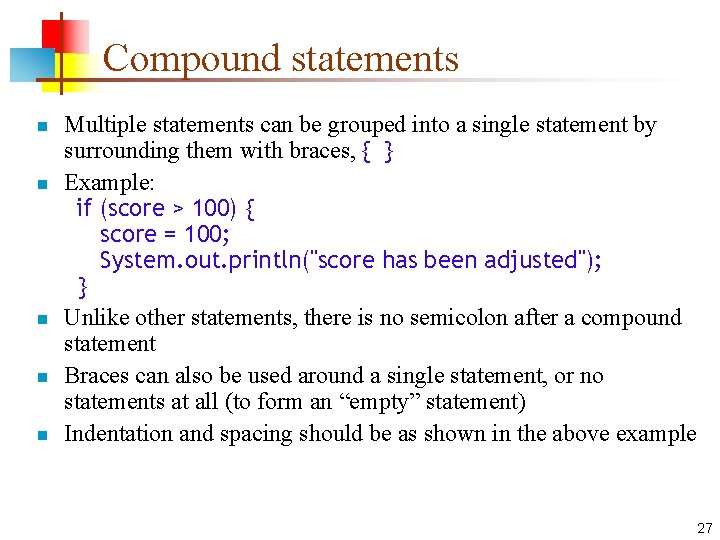Compound statements n n n Multiple statements can be grouped into a single statement by surrounding them with braces, { } Example: if (score > 100) { score = 100; System. out. println("score has been adjusted"); } Unlike other statements, there is no semicolon after a compound statement Braces can also be used around a single statement, or no statements at all (to form an “empty” statement) Indentation and spacing should be as shown in the above example 27while loops n A while loop will execute the enclosed statement as long as a boolean condition remains true n n n Syntax: while (boolean_condition) { statement; } Example: n = 1; while (n < 4) { System. out. println(n + " squared is " + (n * n)); n = n + 1; } Result: 1 squared is 1 2 squared is 4 3 squared is 9 Python programmers: The parentheses are required C programmers: The condition must be boolean Danger: If the condition never becomes false, the loop never exits, and the program never stops 28A complete program n n public class Square. Roots { // Prints the square roots of numbers 1 to 10 public static void main(String args[]) { int n = 1; while (n <= 10) { System. out. println(n + " " + Math. sqrt(n)); n = n + 1; } } } 1 1. 0 2 1. 4142135623730951 3 1. 7320508075688772 4 2. 0 5 2. 23606797749979 etc. 29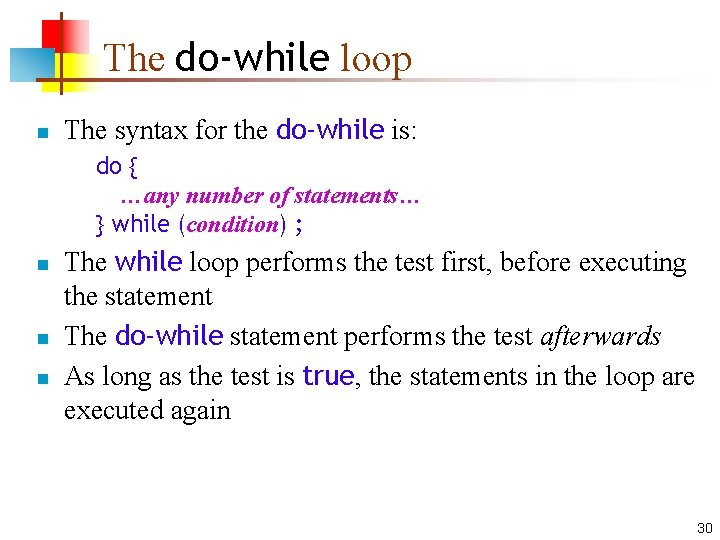The do-while loop n The syntax for the do-while is: do { …any number of statements… } while (condition) ; n n n The while loop performs the test first, before executing the statement The do-while statement performs the test afterwards As long as the test is true, the statements in the loop are executed again 30The increment operator n ++ adds 1 to a variable n n n It can be used as a statement by itself It can be used as part of a larger expression, but this is very bad style (see next slide) It can be put before or after a variable n n n If before a variable (preincrement), it means to add one to the variable, then use the result If put after a variable (postincrement), it means to use the current value of the variable, then add one to the variable When used as a statement, preincrement and postincrement have identical results 31Examples of ++ int a = 5; a++; // a is now 6 int b = 5; ++b; // b is now 6 int c = 5; int d = ++c; // c is 6, d is 6 int e = 5; int f = e++; // e is 6, f is 5 int x = 10; int y = 100; int z = ++x + y++; // x is 11, y is 101, z is 111 Confusing code is bad code, so this is very poor style 32The decrement operator n -- subtracts 1 from a variable n It acts just like ++, and has all the same problems 33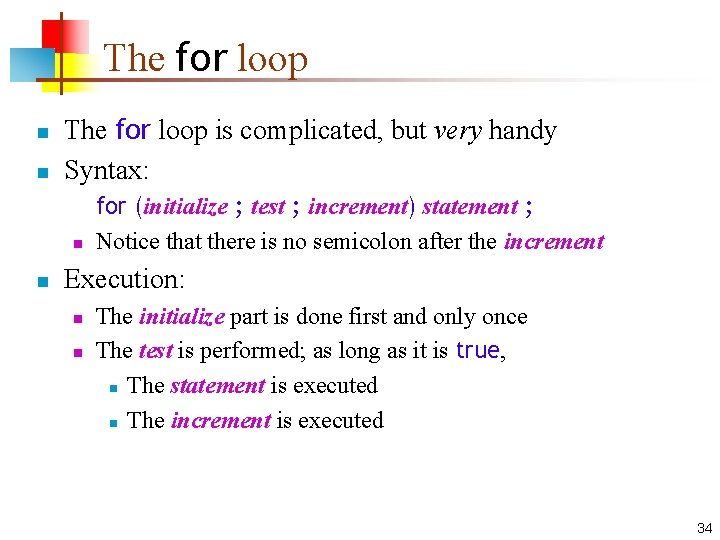The for loop n n The for loop is complicated, but very handy Syntax: n n for (initialize ; test ; increment) statement ; Notice that there is no semicolon after the increment Execution: n n The initialize part is done first and only once The test is performed; as long as it is true, n The statement is executed n The increment is executed 34Parts of the for loop n Initialize: In this part you define the loop variable with an assignment statement, or with a declaration and initialization n n int i = 0, j = k + 1 Test, or condition: A boolean condition n n Examples: i = 0 Just like in the other control statements we have used Increment: An assignment to the loop variable, or an application of ++ or -- to the loop variable n This may be the only good use of ++ and -- ! 35Example for loops n Print the numbers 1 through 10, and their squares: for (int i = 1; i < 11; i++) { System. out. println(i + " " + (i * i)); } n Print the squares of the first 100 integers, ten per line: for (int i = 1; i < 101; i++) { System. out. print(" " + (i * i)); if (i % 10 == 0) System. out. println(); } 36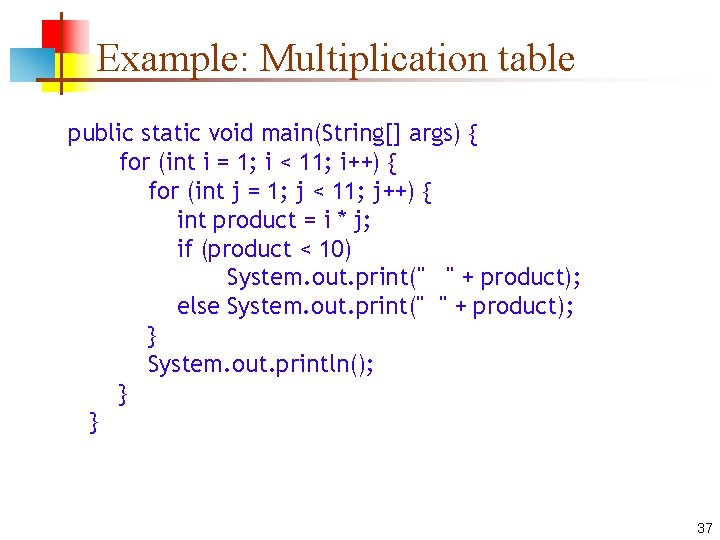Example: Multiplication table public static void main(String[] args) { for (int i = 1; i < 11; i++) { for (int j = 1; j < 11; j++) { int product = i * j; if (product < 10) System. out. print(" " + product); else System. out. print(" " + product); } System. out. println(); } } 37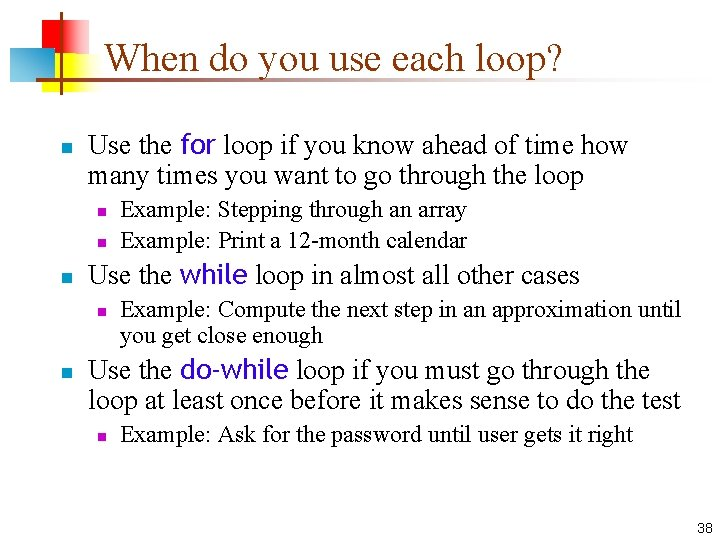When do you use each loop? n Use the for loop if you know ahead of time how many times you want to go through the loop n n n Use the while loop in almost all other cases n n Example: Stepping through an array Example: Print a 12 -month calendar Example: Compute the next step in an approximation until you get close enough Use the do-while loop if you must go through the loop at least once before it makes sense to do the test n Example: Ask for the password until user gets it right 38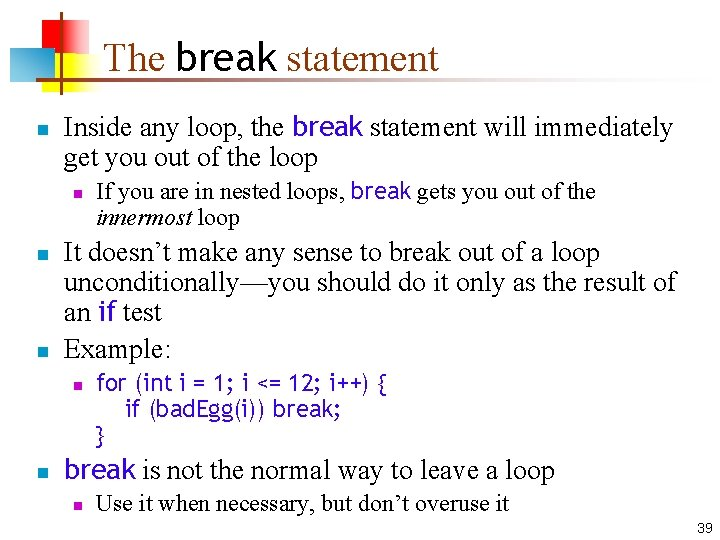The break statement n Inside any loop, the break statement will immediately get you out of the loop n n n It doesn’t make any sense to break out of a loop unconditionally—you should do it only as the result of an if test Example: n n If you are in nested loops, break gets you out of the innermost loop for (int i = 1; i <= 12; i++) { if (bad. Egg(i)) break; } break is not the normal way to leave a loop n Use it when necessary, but don’t overuse it 39The continue statement n Inside any loop, the continue statement will start the next pass through the loop n n In a while or do-while loop, the continue statement will bring you to the test In a for loop, the continue statement will bring you to the increment, then to the test 40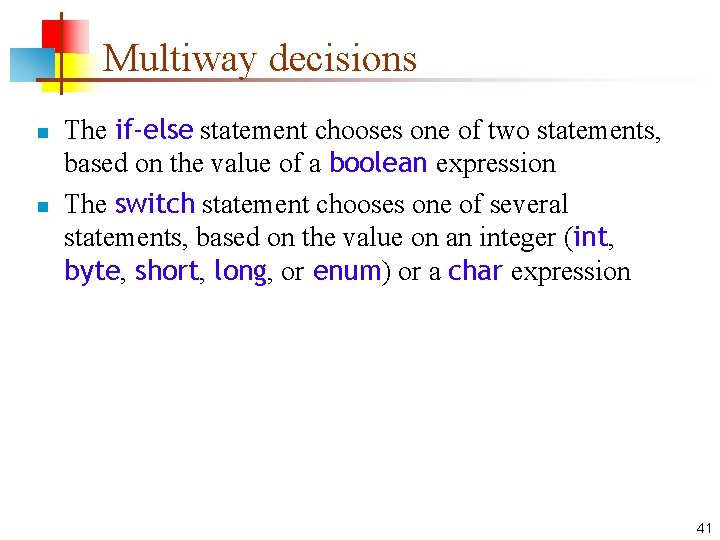Multiway decisions n n The if-else statement chooses one of two statements, based on the value of a boolean expression The switch statement chooses one of several statements, based on the value on an integer (int, byte, short, long, or enum) or a char expression 41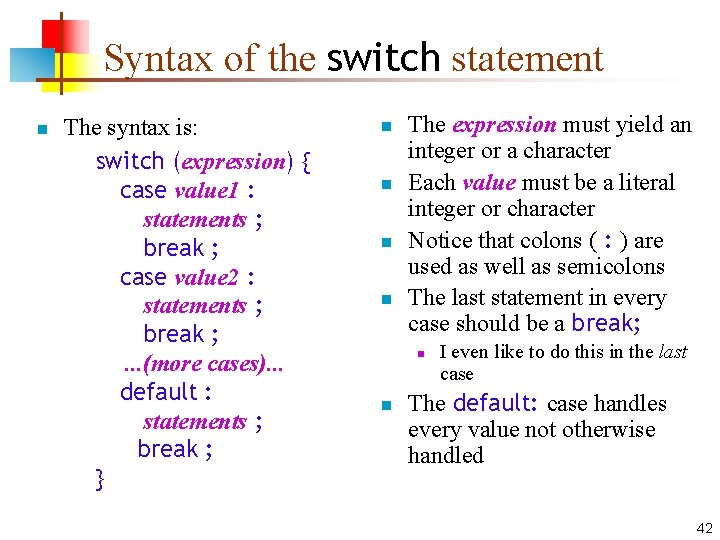Syntax of the switch statement n The syntax is: switch (expression) { case value 1 : statements ; break ; case value 2 : statements ; break ; . . . (more cases). . . default : statements ; break ; } n n The expression must yield an integer or a character Each value must be a literal integer or character Notice that colons ( : ) are used as well as semicolons The last statement in every case should be a break; n n I even like to do this in the last case The default: case handles every value not otherwise handled 42Flowchart for switch statement value statement expression? value statement 43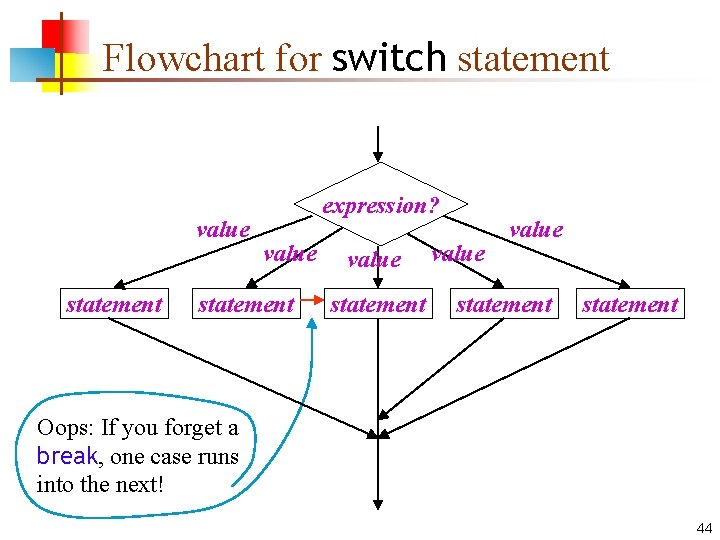Flowchart for switch statement value statement expression? value statement Oops: If you forget a break, one case runs into the next! 44Example switch statement switch (card. Value) { case 1: System. out. print("Ace"); break; case 11: System. out. print("Jack"); break; case 12: System. out. print("Queen"); break; case 13: System. out. print("King"); break; default: System. out. print(card. Value); break; } 45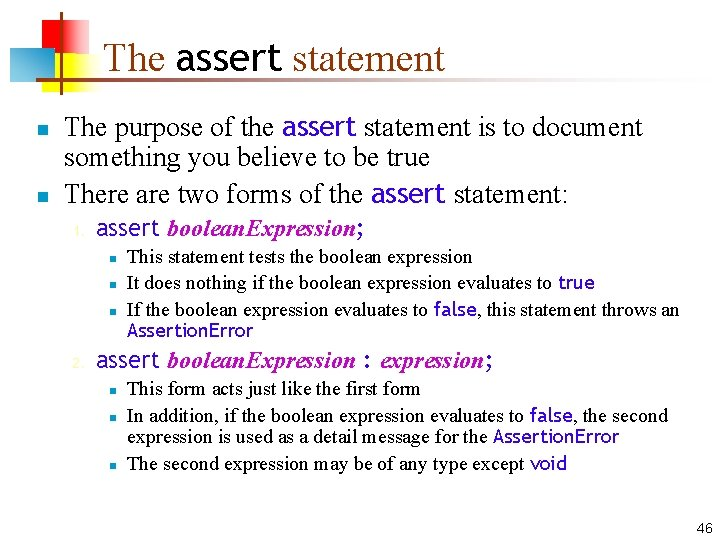The assert statement n n The purpose of the assert statement is to document something you believe to be true There are two forms of the assert statement: 1. assert boolean. Expression; n n n 2. This statement tests the boolean expression It does nothing if the boolean expression evaluates to true If the boolean expression evaluates to false, this statement throws an Assertion. Error assert boolean. Expression : expression; n n n This form acts just like the first form In addition, if the boolean expression evaluates to false, the second expression is used as a detail message for the Assertion. Error The second expression may be of any type except void 46Enabling assertions n By default, Java has assertions disabled—that is, it ignores them n n This is for efficiency Once the program is completely debugged and given to the customer, nothing more will go wrong, so you don’t need the assertions any more n n Yeah, right! You can change this default n n n Open Window Preferences Java Installed JREs Select the JRE you are using (probably 1. 7. something) Click Edit. . . For Default VM Arguments, enter –ea (enable assertions) Click OK (twice) to finish 47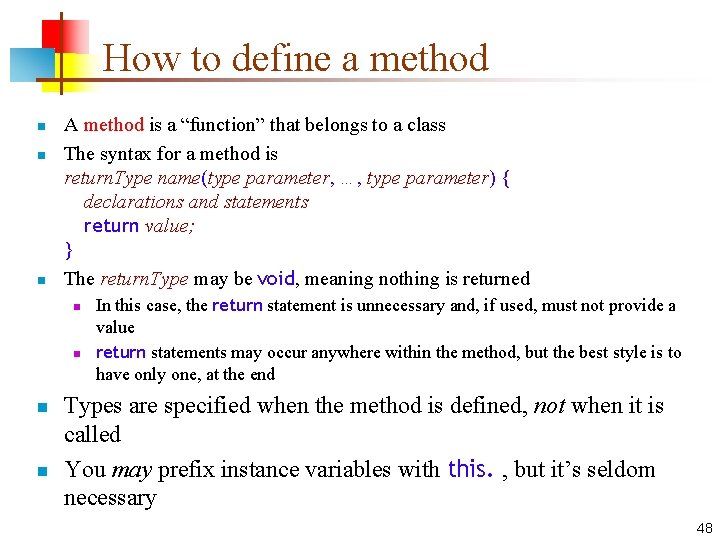How to define a method n n n A method is a “function” that belongs to a class The syntax for a method is return. Type name(type parameter, …, type parameter) { declarations and statements return value; } The return. Type may be void, meaning nothing is returned n n In this case, the return statement is unnecessary and, if used, must not provide a value return statements may occur anywhere within the method, but the best style is to have only one, at the end Types are specified when the method is defined, not when it is called You may prefix instance variables with this. , but it’s seldom necessary 48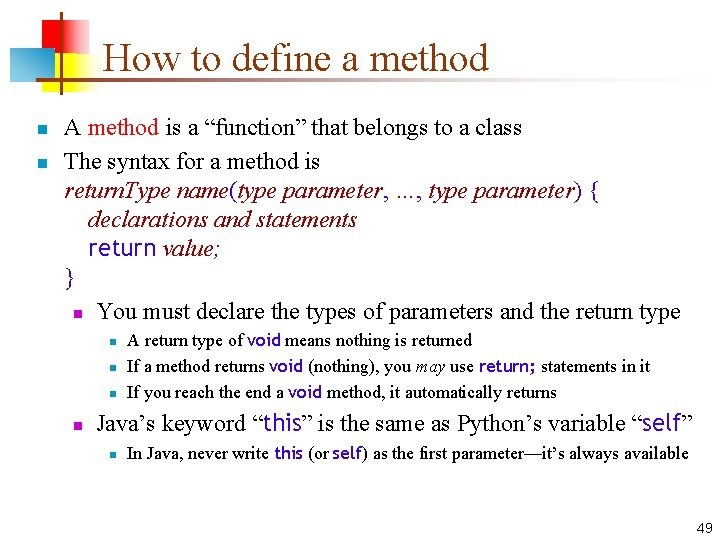How to define a method n n A method is a “function” that belongs to a class The syntax for a method is return. Type name(type parameter, …, type parameter) { declarations and statements return value; } n You must declare the types of parameters and the return type n n A return type of void means nothing is returned If a method returns void (nothing), you may use return; statements in it If you reach the end a void method, it automatically returns Java’s keyword “this” is the same as Python’s variable “self” n In Java, never write this (or self) as the first parameter—it’s always available 49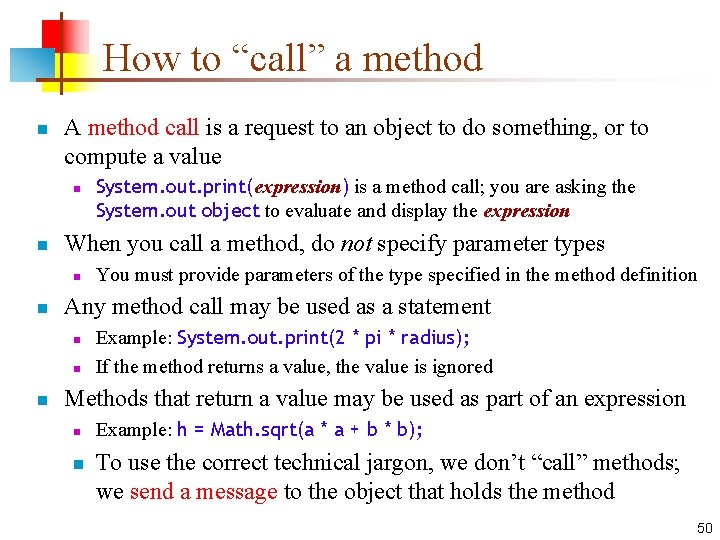How to “call” a method n A method call is a request to an object to do something, or to compute a value n n When you call a method, do not specify parameter types n n You must provide parameters of the type specified in the method definition Any method call may be used as a statement n n n System. out. print(expression) is a method call; you are asking the System. out object to evaluate and display the expression Example: System. out. print(2 * pi * radius); If the method returns a value, the value is ignored Methods that return a value may be used as part of an expression n n Example: h = Math. sqrt(a * a + b * b); To use the correct technical jargon, we don’t “call” methods; we send a message to the object that holds the method 50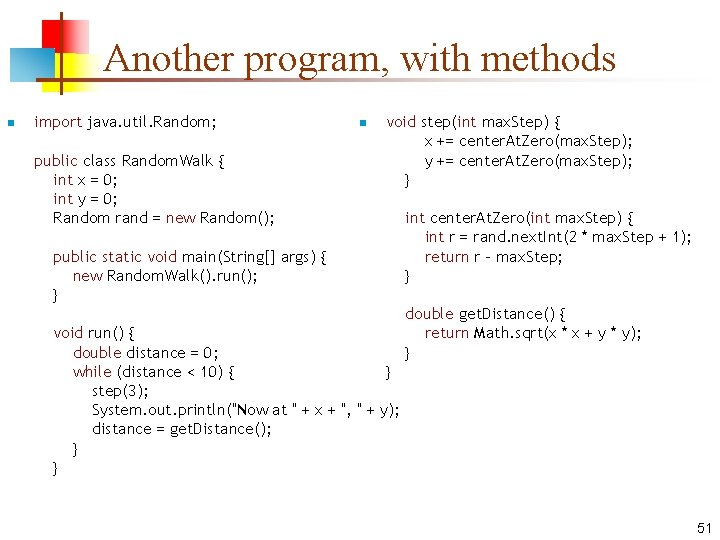Another program, with methods n import java. util. Random; public class Random. Walk { int x = 0; int y = 0; Random rand = new Random(); n void step(int max. Step) { x += center. At. Zero(max. Step); y += center. At. Zero(max. Step); } public static void main(String[] args) { new Random. Walk(). run(); } void run() { double distance = 0; while (distance < 10) { } step(3); System. out. println("Now at " + x + ", " + y); distance = get. Distance(); } } int center. At. Zero(int max. Step) { int r = rand. next. Int(2 * max. Step + 1); return r - max. Step; } double get. Distance() { return Math. sqrt(x * x + y * y); } 51The End “I think there is a world market for maybe five computers. ” —Thomas Watson, Chairman of IBM, 1943 “There is no reason anyone would want a computer in their home. ” —Ken Olsen, president/founder of Digital Equipment Corporation, 1977 52